### Computational Problems

#### First Iteration Overflows

Analyzing a covariance matrix that includes high variances in the diagonal and using bad initial estimates for the parameters can easily lead to arithmetic overflows in the first iterations of the minimization algorithm. The line-search algorithms that work with cubic extrapolation are especially sensitive to arithmetic overflows. If this occurs with quasi-Newton or conjugate gradient minimization, you can specify the INSTEP= option to reduce the length of the first step. If an arithmetic overflow occurs in the first iteration of the Levenberg-Marquardt algorithm, you can specify the INSTEP= option to reduce the trust region radius of the first iteration. You also can change the minimization technique or the line-search method. If none of these help, you can consider doing the following:

• scaling the covariance matrix

• providing better initial values

• changing the model

#### No Convergence of Minimization Process

If convergence does not occur during the minimization process, perform the following tasks:

• If there are negative variance estimates, you can do either of the following:

• Specify the BOUNDS statement to obtain nonnegative variance estimates.

• Specify the HEYWOOD option, if the FACTOR statement is specified.

• Change the estimation method to obtain a better set of initial estimates. For example, if you use METHOD=ML, you can do either of the following:

• Change the optimization technique. For example, if you use the default OMETHOD=LEVMAR, you can do either of the following:

• Change to OMETHOD=QUANEW or to OMETHOD=NEWRAP.

• Run some iterations with OMETHOD=CONGRA, write the results in an OUTMODEL= data set, and use the results as initial values specified by an INMODEL= data set in a second run with a different OMETHOD= technique.

• Change or modify the update technique or the line-search algorithm or both when using OMETHOD=QUANEW or OMETHOD=CONGRA. For example, if you use the default update formula and the default line-search algorithm, you can do any or all of the following:

• Change the update formula with the UPDATE= option.

• Change the line-search algorithm with the LIS= option.

• Specify a more precise line search with the LSPRECISION= option, if you use LIS=2 or LIS=3.

• Add more iterations and function calls by using the MAXIT= and MAXFU= options.

• Change the initial values. For many categories of model specifications, PROC CALIS computes an appropriate set of initial values automatically. However, for some of the model specifications (for example, structural equations with latent variables on the left-hand side and manifest variables on the right-hand side), PROC CALIS might generate very obscure initial values. In these cases, you have to set the initial values yourself.

• Increase the initial values of the variance parameters by one of the following ways:

• Set the variance parameter values in the model specification manually.

• Use the DEMPHAS= option to increase all initial variance parameter values.

• Use a slightly different, but more stable, model to obtain preliminary estimates.

• Use additional information to specify initial values, for example, by using other SAS software like the FACTOR, REG, SYSLIN, and MODEL (SYSNLIN) procedures for the modified, unrestricted model case.

#### Unidentified Model

The parameter vector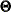in the structural model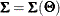is said to be identified in a parameter space G, if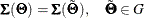implies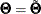. The parameter estimates that result from an unidentified model can be very far from the parameter estimates of a very similar but identified model. They are usually machine dependent. Do not use parameter estimates of an unidentified model as initial values for another run of PROC CALIS.

#### Singular Predicted Covariance Model Matrix

Sometimes you might inadvertently specify models with singular predicted covariance model matrices (for example, by fixing diagonal elements to zero). In such cases, you cannot compute maximum likelihood estimates (the ML function value F is not defined). Since singular predicted covariance model matrices can also occur temporarily in the minimization process, PROC CALIS tries in such cases to change the parameter estimates so that the predicted covariance model matrix becomes positive definite. This process does not always work well, especially if there are fixed instead of free diagonal elements in the predicted covariance model matrices. A famous example where you cannot compute ML estimates is a component analysis with fewer components than given manifest variables. See the section FACTOR Statement for more details. If you continue to obtain a singular predicted covariance model matrix after changing initial values and optimization techniques, then your model might be specified so that ML estimates cannot be computed.

#### Saving Computing Time

For large models, the most computing time is needed to compute the modification indices. If you do not really need the Lagrange multipliers or multiple Wald test indices (the univariate Wald test indices are the same as the t values), using the NOMOD option can save a considerable amount of computing time.

#### Predicted Covariance Matrices with Negative Eigenvalues

A covariance matrix cannot have negative eigenvalues, since a negative eigenvalue means that some linear combination of the variables has negative variance. PROC CALIS displays a warning if the predicted covariance matrix has negative eigenvalues but does not actually compute the eigenvalues. Sometimes this warning can be triggered by 0 or very small positive eigenvalues that appear negative because of numerical error. If you want to be sure that the predicted covariance matrix you are fitting can be considered to be a variance-covariance matrix, you can use the SAS/IML command VAL=EIGVAL(U) to compute the vector VAL of eigenvalues of matrix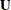.

#### Negative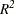Values

The estimated squared multiple correlations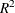of the endogenous variables are computed using the estimated error variances: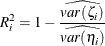When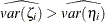,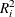is negative. This might indicate poor model fit or R square is an inappropriate measure for the model. For the latter case, for example, negative R square might be due to cyclical (nonrecursive) paths in the model so that the R square interpretation is not appropriate.